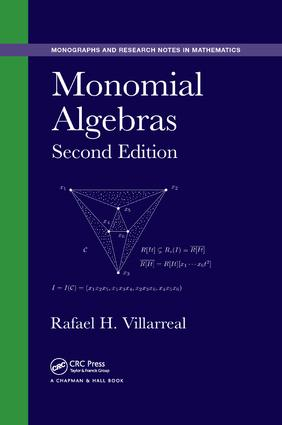Monomial Algebras

2nd Edition

Chapman and Hall/CRC

704 pages | 106 B/W Illus.

Purchasing Options:\$ = USD
Paperback: 9781138894181
pub: 2018-03-12
SAVE ~\$16.59
\$82.95
\$66.36
x
Hardback: 9781482234695
pub: 2015-03-26
SAVE ~\$42.00
\$210.00
\$168.00
x
eBook (VitalSource) : 9781351229203
pub: 2018-10-08
from \$41.48

FREE Standard Shipping!

Description

Monomial Algebras, Second Edition presents algebraic, combinatorial, and computational methods for studying monomial algebras and their ideals, including Stanley–Reisner rings, monomial subrings, Ehrhart rings, and blowup algebras. It emphasizes square-free monomials and the corresponding graphs, clutters, or hypergraphs.

New to the Second Edition

• Four new chapters that focus on the algebraic properties of blowup algebras in combinatorial optimization problems of clutters and hypergraphs
• Two new chapters that explore the algebraic and combinatorial properties of the edge ideal of clutters and hypergraphs
• Full revisions of existing chapters to provide an up-to-date account of the subject

Bringing together several areas of pure and applied mathematics, this book shows how monomial algebras are related to polyhedral geometry, combinatorial optimization, and combinatorics of hypergraphs. It directly links the algebraic properties of monomial algebras to combinatorial structures (such as simplicial complexes, posets, digraphs, graphs, and clutters) and linear optimization problems.

Reviews

"… an introduction to algebraic, combinatorial, and computational aspects of monomial ideals. In the second edition, a full revision of all the chapters has been made."

Zentralblatt MATH 1325

Polyhedral Geometry and Linear Optimization

Polyhedral sets and cones

Relative volumes of lattice polytopes

Hilbert bases and TDI systems

Rees cones and clutters

The integral closure of a semigroup

Unimodularity of matrices and normality

Normaliz, a computer program

Cut-incidence matrices and integrality

Elementary vectors and matroids

Commutative Algebra

Module theory

Graded modules and Hilbert polynomials

Cohen–Macaulay modules

Normal rings

Valuation rings

Krull rings

Koszul homology

A vanishing theorem of Grothendieck

Affine and Graded Algebras

Hilbert Nullstellensatz

Gröbner bases

Projective closure

Minimal resolutions

Rees Algebras and Normality

Symmetric algebras

Rees algebras and syzygetic ideals

Complete and normal ideals

Multiplicities and a criterion of Herzog

Jacobian criterion

Hilbert Series

Hilbert–Serre Theorem

a-invariants and h-vectors

Extremal algebras

Initial degrees of Gorenstein ideals

Koszul homology and Hilbert functions

Hilbert functions of some graded ideals

Stanley–Reisner Rings and Edge Ideals of Clutters

Primary decomposition

Simplicial complexes and homology

Stanley–Reisner rings

Regularity and projective dimension

Unmixed and shellable clutters

Hilbert series of face rings

Simplicial spheres

The upper bound conjectures

Edge Ideals of Graphs

Graph theory

Edge ideals and B-graphs

Cohen–Macaulay and chordal graphs

Shellable and sequentially C–M graphs

Regularity, depth, arithmetic degree

Betti numbers of edge ideals

Associated primes of powers of ideals

Toric Ideals and Affine Varieties

Binomial ideals and their radicals

Lattice ideals

Monomial subrings and toric ideals

Toric varieties

Affine Hilbert functions

Vanishing ideals over finite fields

Semigroup rings of numerical semigroups

Toric ideals of monomial curves

Monomial Subrings

Integral closure of monomial subrings

Homogeneous monomial subrings

Ehrhart rings

The degree of lattice and toric ideals

Laplacian matrices and ideals

Gröbner bases and normal subrings

Toric ideals generated by circuits

Divisor class groups of semigroup rings

Monomial Subrings of Graphs

Edge subrings and ring graphs

Incidence matrices and circuits

The integral closure of an edge subring

Ehrhart rings of edge polytopes

Integral closure of Rees algebras

Edge subrings of complete graphs

Edge cones of graphs

Monomial birational extensions

Edge Subrings and Combinatorial Optimization

The canonical module of an edge subring

Integrality of the shift polyhedron

Generators for the canonical module

Computing the a-invariant

Algebraic invariants of edge subrings

Normality of Rees Algebras of Monomial Ideals

Integral closure of monomial ideals

Normality criteria

Rees cones and polymatroidal ideals

Veronese subrings and the a-invariant

Normalizations of Rees algebras

Rees algebras of Veronese ideals

Divisor class group of a Rees algebra

Stochastic matrices and Cremona maps

Combinatorics of Symbolic Rees Algebras of Edge Ideals of Clutters

Vertex covers of clutters

Symbolic Rees algebras of edge ideals

Blowup algebras in perfect graphs

Algebras of vertex covers of graphs

Edge subrings in perfect matchings

Rees cones and perfect graphs

Perfect graphs and algebras of covers

Combinatorial Optimization and Blowup Algebras

Blowup algebras of edge ideals

Rees algebras and polyhedral geometry

Packing problems and blowup algebras

Uniform ideal clutters

Clique clutters of comparability graphs

Duality and integer rounding problems

Canonical modules and integer rounding

Clique clutters of Meyniel graphs

Appendix: Graph Diagrams

Bibliography

Notation Index

Index

Dr. Rafael H. Villarreal is a professor in the Department of Mathematics at the Centro de Investigación y de Estudios Avanzados del I.P.N. (Cinvestav-IPN). His research focuses on commutative algebra, algebraic geometry, combinatorics, and computational algebra.